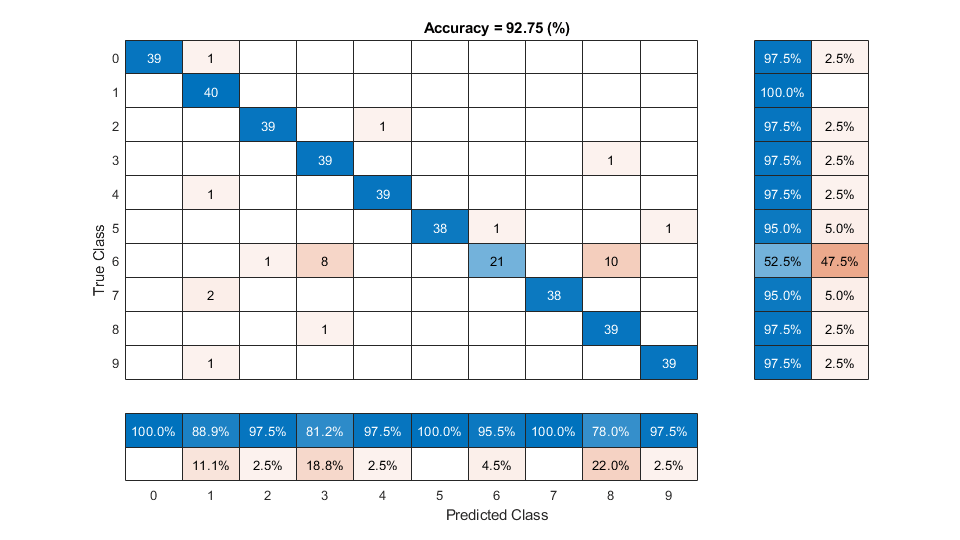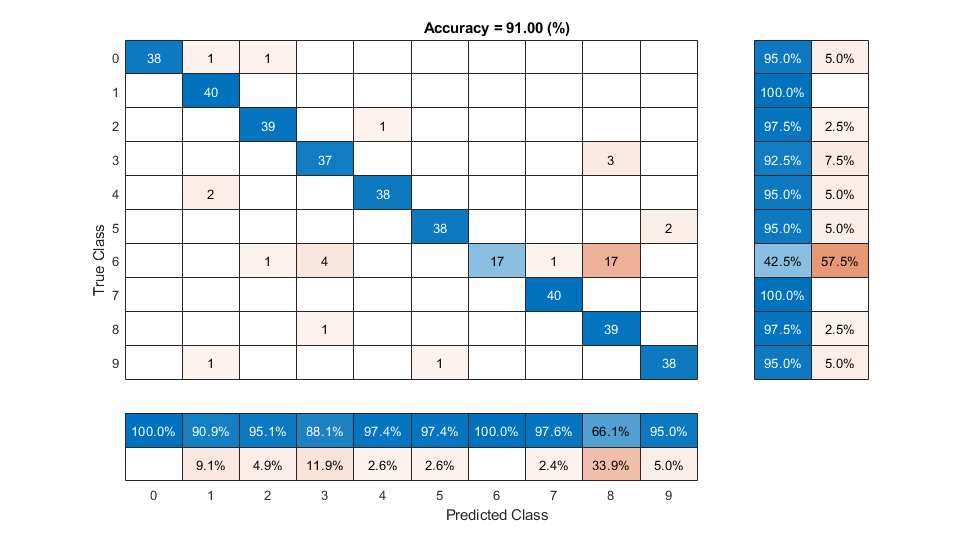# ivector

Extract i-vector

## Syntax

``w = ivector(ivs,data)``
``w = ivector(ivs,data,Name,Value)``

## Description

example

````w = ivector(ivs,data)` extracts i-vectors from the input `data`.```
````w = ivector(ivs,data,Name,Value)` specifies additional options using name-value arguments. You can choose the hardware resource for extracting i-vectors and whether to apply the projection matrix from `trainClassifier`.```

## Examples

collapse all

An i-vector system consists of a trainable front end that learns how to extract i-vectors based on unlabeled data, and a trainable backend that learns how to classify i-vectors based on labeled data. In this example, you apply an i-vector system to the task of word recognition. First, evaluate the accuracy of the i-vector system using the classifiers included in a traditional i-vector system: probabilistic linear discriminant analysis (PLDA) and cosine similarity scoring (CSS). Next, evaluate the accuracy of the system if you replace the classifier with bidirectional long short-term memory (BiLSTM) network or a K-nearest neighbors classifier.

Create Training and Validation Sets

Download the Free Spoken Digit Dataset (FSDD) . FSDD consists of short audio files with spoken digits (0-9).

```loc = matlab.internal.examples.downloadSupportFile("audio","FSDD.zip"); unzip(loc,pwd)```

Create an `audioDatastore` to point to the recordings. Get the sample rate of the data set.

```ads = audioDatastore(pwd,IncludeSubfolders=true); [~,adsInfo] = read(ads); fs = adsInfo.SampleRate;```

The first element of the file names is the digit spoken in the file. Get the first element of the file names, convert them to categorical, and then set the `Labels` property of the `audioDatastore`.

```[~,filenames] = cellfun(@(x)fileparts(x),ads.Files,UniformOutput=false); ads.Labels = categorical(string(cellfun(@(x)x(1),filenames)));```

To split the datastore into a development set and a validation set, use `splitEachLabel`. Allocate 80% of the data for development and the remaining 20% for validation.

`[adsTrain,adsValidation] = splitEachLabel(ads,0.8);`

Create an i-vector system that expects audio input at a sample rate of 8 kHz and does not perform speech detection.

`wordRecognizer = ivectorSystem(DetectSpeech=false,SampleRate=fs)`
```wordRecognizer = ivectorSystem with properties: InputType: 'audio' SampleRate: 8000 DetectSpeech: 0 Verbose: 1 EnrolledLabels: [0×2 table] ```

Train the i-vector extractor using the data in the training set.

```trainExtractor(wordRecognizer,adsTrain, ... UBMNumComponents=64, ... UBMNumIterations=5, ... ... TVSRank=32, ... TVSNumIterations=5);```
```Calculating standardization factors ....done. Training universal background model ........done. Training total variability space ........done. i-vector extractor training complete. ```

Train the i-vector classifier using the data in the training data set and the corresponding labels.

```trainClassifier(wordRecognizer,adsTrain,adsTrain.Labels, ... NumEigenvectors=10, ... ... PLDANumDimensions=10, ... PLDANumIterations=5);```
```Extracting i-vectors ...done. Training projection matrix .....done. Training PLDA model ........done. i-vector classifier training complete. ```

Calibrate the scores output by `wordRecognizer` so they can be interpreted as a measure of confidence in a positive decision. Enroll labels into the system using the entire training set.

`calibrate(wordRecognizer,adsTrain,adsTrain.Labels)`
```Extracting i-vectors ...done. Calibrating CSS scorer ...done. Calibrating PLDA scorer ...done. Calibration complete. ```
`enroll(wordRecognizer,adsTrain,adsTrain.Labels)`
```Extracting i-vectors ...done. Enrolling i-vectors .............done. Enrollment complete. ```

In a loop, read audio from the validation datastore, identify the most-likely word present according to the specified scorer, and save the prediction for analysis.

```trueLabels = adsValidation.Labels; predictedLabels = trueLabels; reset(adsValidation) scorer ="plda"; for ii = 1:numel(trueLabels) audioIn = read(adsValidation); to = identify(wordRecognizer,audioIn,scorer); predictedLabels(ii) = to.Label(1); end```

Display a confusion chart of the i-vector system's performance on the validation set.

```figure(Units="normalized",Position=[0.2 0.2 0.5 0.5]) confusionchart(trueLabels,predictedLabels, ... ColumnSummary="column-normalized", ... RowSummary="row-normalized", ... Title=sprintf('Accuracy = %0.2f (%%)',100*mean(predictedLabels==trueLabels)))```Evaluate Deep Learning Backend Performance

Next, train a fully-connected network using i-vectors as input.

```ivectorsTrain = (ivector(wordRecognizer,adsTrain))'; ivectorsValidation = (ivector(wordRecognizer,adsValidation))';```

Define a fully connected network.

```layers = [ ... featureInputLayer(size(ivectorsTrain,2),Normalization="none") fullyConnectedLayer(128) dropoutLayer(0.4) fullyConnectedLayer(256) dropoutLayer(0.4) fullyConnectedLayer(256) dropoutLayer(0.4) fullyConnectedLayer(128) dropoutLayer(0.4) fullyConnectedLayer(numel(unique(adsTrain.Labels))) softmaxLayer classificationLayer];```

Define training parameters.

```miniBatchSize = 256; validationFrequency = floor(numel(adsTrain.Labels)/miniBatchSize); options = trainingOptions("adam", ... MaxEpochs=10, ... MiniBatchSize=miniBatchSize, ... Plots="training-progress", ... Verbose=false, ... Shuffle="every-epoch", ... ValidationData={ivectorsValidation,adsValidation.Labels}, ... ValidationFrequency=validationFrequency);```

Train the network.

`net = trainNetwork(ivectorsTrain,adsTrain.Labels,layers,options);`Evaluate the performance of the deep learning backend using a confusion chart.

```predictedLabels = classify(net,ivectorsValidation); trueLabels = adsValidation.Labels; figure(Units="normalized",Position=[0.2 0.2 0.5 0.5]) confusionchart(trueLabels,predictedLabels, ... ColumnSummary="column-normalized", ... RowSummary="row-normalized", ... Title=sprintf('Accuracy = %0.2f (%%)',100*mean(predictedLabels==trueLabels)))```Evaluate KNN Backend Performance

Train and evaluate i-vectors with a k-nearest neighbor (KNN) backend.

Use `fitcknn` to train a KNN model.

```classificationKNN = fitcknn(... ivectorsTrain, ... adsTrain.Labels, ... Distance="Euclidean", ... Exponent=[], ... NumNeighbors=10, ... DistanceWeight="SquaredInverse", ... Standardize=true, ... ClassNames=unique(adsTrain.Labels));```

Evaluate the KNN backend.

```predictedLabels = predict(classificationKNN,ivectorsValidation); trueLabels = adsValidation.Labels; figure(Units="normalized",Position=[0.2 0.2 0.5 0.5]) confusionchart(trueLabels,predictedLabels, ... ColumnSummary="column-normalized", ... RowSummary="row-normalized", ... Title=sprintf('Accuracy = %0.2f (%%)',100*mean(predictedLabels==trueLabels)))```References

 Jakobovski. "Jakobovski/Free-Spoken-Digit-Dataset." GitHub, May 30, 2019. `https://github.com/Jakobovski/free-spoken-digit-dataset`.

## Input Arguments

collapse all

i-vector system, specified as an object of type `ivectorSystem`.

Data to transform, specified as a cell array or as an `audioDatastore`, `signalDatastore`, or `TransformedDatastore` object.

• If `InputType` is set to `"audio"` when the i-vector system is created, specify `data` as one of these:

• If `InputType` is set to `"features"` when the i-vector system is created, specify `data` as one of these:

• A matrix with underlying type `single` or `double`. The matrix must consist of audio features where the number of features (columns) is locked the first time `trainExtractor` is called and the number of hops (rows) is variable-sized. The number of features input in any subsequent calls to any of the object functions must be equal to the number of features used when calling `trainExtractor`.

• A cell array of matrices with underlying type `single` or `double`. The matrices must consist of audio features where the number of features (columns) is locked the first time `trainExtractor` is called and the number of hops (rows) is variable-sized. The number of features input in any subsequent calls to any of the object functions must be equal to the number of features used when calling `trainExtractor`.

• A `TransformedDatastore` object with an underlying `audioDatastore` or `signalDatastore` whose `read` function has output as described in the previous bullet.

• A `signalDatastore` object whose `read` function has output as described in the first bullet.

Data Types: `cell` | `audioDatastore` | `signalDatastore`

### Name-Value Arguments

Specify optional pairs of arguments as `Name1=Value1,...,NameN=ValueN`, where `Name` is the argument name and `Value` is the corresponding value. Name-value arguments must appear after other arguments, but the order of the pairs does not matter.

Before R2021a, use commas to separate each name and value, and enclose `Name` in quotes.

Example: `ivector(ivs,data,ApplyProjectionMatrix=false,ExecutionEnvironment="parallel")`

Option to apply projection matrix, specified as a logical value. This argument specifies whether to apply the linear discriminant analysis (LDA) and within-class covariance normalization (WCCN) projection matrix determined using `trainClassifier`.

• If the projection matrix was trained, then `ApplyProjectionMatrix` defaults to `true`.

• If the projection matrix was not trained, then `ApplyProjectionMatrix` defaults to `false` and cannot be set to `true`.

Data Types: `logical`

Hardware resource for execution, specified as one of these:

• `"auto"` — Use the GPU if it is available. Otherwise, use the CPU.

• `"cpu"` — Use the CPU.

• `"gpu"` — Use the GPU. This option requires Parallel Computing Toolbox™.

• `"multi-gpu"` — Use multiple GPUs on one machine, using a local parallel pool based on your default cluster profile. If there is no current parallel pool, the software starts a parallel pool with pool size equal to the number of available GPUs. This option requires Parallel Computing Toolbox.

• `"parallel"` — Use a local or remote parallel pool based on your default cluster profile. If there is no current parallel pool, the software starts one using the default cluster profile. If the pool has access to GPUs, then only workers with a unique GPU perform training computation. If the pool does not have GPUs, then the training takes place on all available CPU workers. This option requires Parallel Computing Toolbox.

Data Types: `char` | `string`

Option to use prefetch queuing when reading from a datastore, specified as a logical value. This argument requires Parallel Computing Toolbox.

Data Types: `logical`

## Output Arguments

collapse all

Extracted i-vectors, returned as a column vector or a matrix. The number of columns of `w` is equal to the number of input signals. The number of rows of `w` is the dimension of the i-vector.

## Version History

Introduced in R2021a